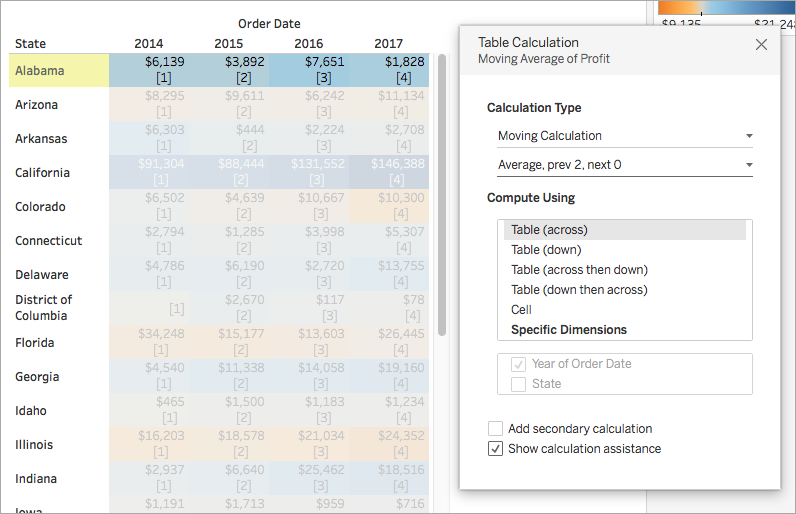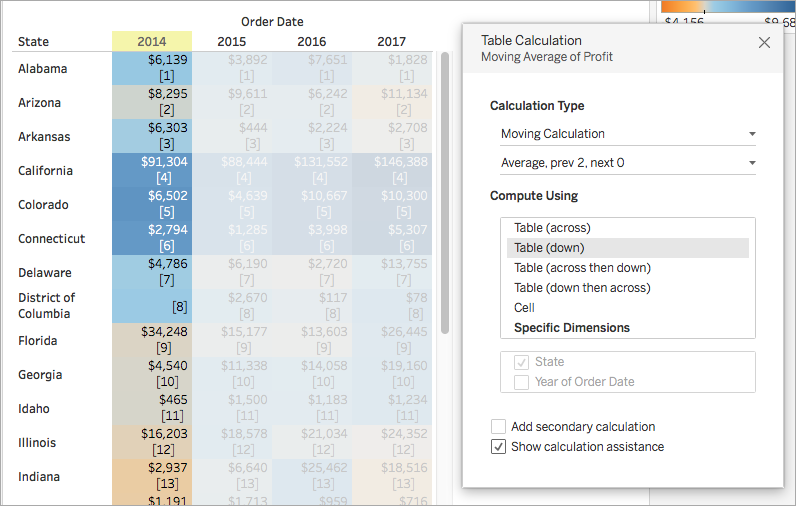# Quick Table Calculations

Quick table calculations allow you to quickly apply a common table calculation to your visualization using the most typical settings for that calculation type. This article demonstrates how to apply a quick table calculation to a visualization using an example.

The following quick table calculations are available in Tableau for you to use:

• Running total
• Difference
• Percent difference
• Percent of total
• Rank
• Percentile
• Moving average
• YTD total
• Compound growth rate
• Year of year growth
• YTD growth

## How does a quick table calculation differ from a table calculation?

Quick table calculations are table calculations that you can apply quickly to your visualization in Tableau. They are applied to the visualization with the most typical settings for the calculation type you choose so that you can continue on with your analysis. With traditional table calculations, you can apply the same settings, but you must apply them manually.

## Apply a quick table calculation to the visualization

Follow along with the steps below to learn how to apply a quick table calculation to a visualization.

### Set up the visualization

1. Open Tableau Desktop and connect to the Sample-Superstore data source, which comes with Tableau and navigate to a new worksheet.
2. From the Data pane, drag Order Date to the Columns shelf.
3. Drag State to the Rows shelf.
4. Drag Sales to Text on the Marks Card.
5. Drag Profit to Color on the Marks Card.
6. On the Marks card, click the Mark Type drop-down and select Square.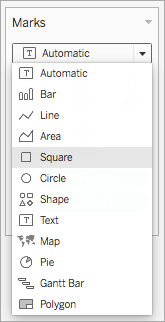The visualization updates to look like this: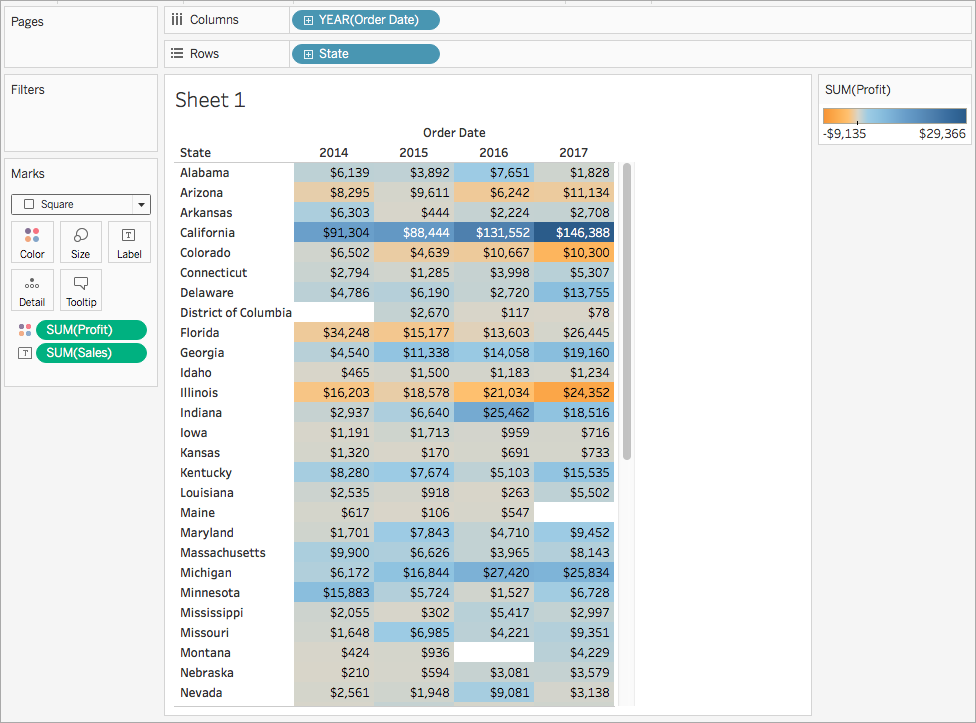### Apply the quick table calculation

1. On the Marks card, right-click SUM(Profit) and select Quick Table Calculation > Moving Average.

Note: You can only perform quick table calculations on measures in the view.

A delta symbol appears on the field to indicate that a quick table calculation is being applied to the field. The colors in the visualization update to show the moving average of profit across the years.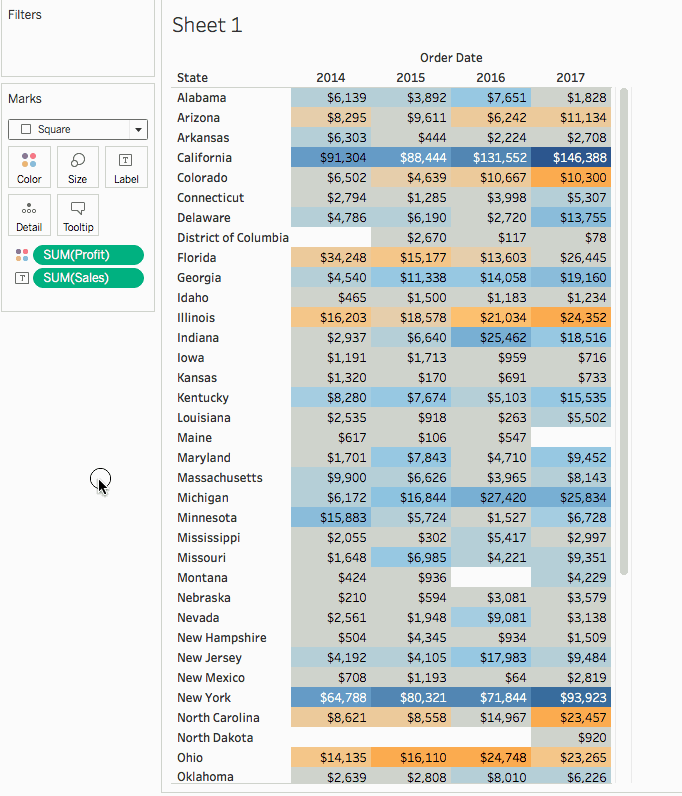### (Optional) Customize the quick table calculation

1. On the Marks card, right-click Sum(Profit) and select Edit Table Calculation.

2. In the dialog box that opens, you can configure the following options:

• The calculation type
• How to aggregate the values
• How to compute the calculation (how to address and partition the calculation)